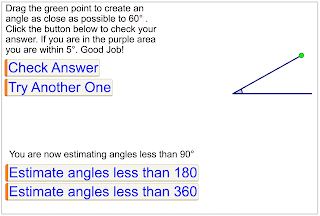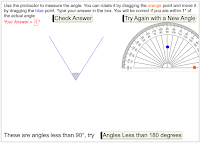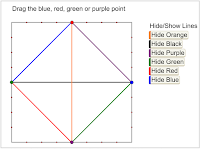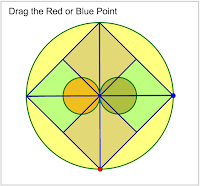## Pages

### Dynamic Web Sketches

I started to turn my Geometer's Sketchpad into WebSketches. This makes it possible to use any of these dynamic sketches without the GSP software. So even though you could use the Sketchpad Explorer app for iPads, with these links you could also use them on a Chromebook (or any other web enabled device). In most cases there is a link to the blog post with the complete instructions as well as a link to the original GSP sketch. A complete list of my original sketches can be found here.## Rotation Investigation

Web sketch here
Original Sketch here
When students begin to describe rotations it's helpful to do so by only considering rotational angles in multiples of 90 degrees. In this series of websketches students start by investigating rotation of a hexagon and then an irregular shape. Finally students are shown random rotations and asked to mimic them for both hexagons and irregular shapes. For a brief description on how to use it you can see this video.
Curriculum Tags: Gr6G, Gr7G, Gr8G## Cosine Law Practice

Web sketch here
Original Sketch here
This sketch starts out by asking students to verify that cosine law works for any triangle. Then they can solve for a side or an angle by generating random triangles. Full solutions for each random triangle is shown.
Curriculum Tags: MPM2D, MCF3M, MBF3C, MCR3U## Sine Law Practice

Web sketch here
Original Sketch here
This sketch starts out by asking students to verify that sine law works for any triangle. Then they can solve for a side or an angle by generating random triangles. Full solutions for each random triangle is shown.
Curriculum Tags: MPM2D, MCF3M, MBF3C, MCR3U## Trapezoid Area

Web sketch here
Original Sketch here
This is a demonstration sketch that shows how universal the formula for the area of a trapezoid is. That is, by using that formula, you can also calculate the area of a parallelogram, rhombus, rectangle, square and even a triangle. For a video explanation you can also look here.
Curriculum Tags: Gr7M## Estimating Angles

Web sketch here
Original Sketch here
This sketch lets students estimate the size of a given angle value. They are randomly given an angle and then have to move the arm to create an angle of that size. They can check their answers and then randomly generate another angle. Students can estimate angles less than 90o, 180o or 360o.
Curriculum Tags: Gr6G## Equation Strips

Web sketch here
Original Sketch here
This is a utility sketch for teachers for dealing with solving simple one and two step equations using a visual representation. The purpose of this sketch is to be able to dynamically create Equations strips for equations in the form ax + b = c where a, b & c are natural numbers (though now I've added a page for equations in the form ax - b = c). Once you create your equation, use the screen capture software of your choice to copy and paste the version you want to use (For Windows use the Snipping Tool, for Chromebooks use Shift CTRL F5, for Macs use Command Shift 4, or iPad use the Home and Sleep buttons together .
Curriculum Tags: Gr7PA, Gr8PA, MFM1P## Rotations

Web sketch here
Original Sketch here
This is a practice sketch for dealing with rotation of points. In this case, the vertices of a triangle. The first two pages lets students just drag a single point to rotate the shape. Then they have to drag individual points. On the next page they use the coordinates to show the image. And then finally they can create their own question.
Curriculum Tags: Gr6G, Gr7G, Gr8G## Translations

Web sketch here
Original Sketch here
This is a practice sketch for dealing with translation of points. In this case, the vertices of a triangle. The first page lets students just drag points to show the image. On the next page they use the coordinates to show the image. And then finally they can create their own question.
Curriculum Tags: Gr6G, Gr7G, Gr8G## Pythagorean Area

Web sketch here
Original Sketch here
We know that the area of the smaller squares on a right angle triangle sum to equal the area of the larger square. But it turns out that that works for other shapes besides squares. It even works for irregularly shaped objects too. Or does it? Investigate that with this web sketch. For a more involved activity you may wish to try this one.
Curriculum Tags: Gr8M, MFM1P

## Order of Rotational Symmetry

Web sketch here
Original Sketch here
This sketch was based on a paper and pencil problem that a friend of mine showed me. The idea is that students can show or hide any of the shading leaves then rotate the figure to see how many times it looks the same in one rotation (the order of rotational symmetry)
Curriculum Tags: Gr6G

Web sketch here
Original Sketch here
This sketch dynamically models a classic calculus problem: a ladder where the bottom slides away from the wall. In this sketch they can move the ladder manually or automatically.
Curriculum Tags: MCV4U## Measuring Angles Practice

Web sketch here
Original Sketch here
In this sketch students can practice measuring angles with a protractor. They can measure angles less than 90o, 180o or 360o. When they check their answer they will be marked correct if they are within one degree. They can then generate an infinite number of new angles to measure.
Curriculum Tags: Gr6G## Trapezoid/Triangle Area

Web sketch here
Original Sketch here
In the last couple of months I have seen a couple of videos of this transformation from trapezoid to triangle to prove the formula for the area of a trapezoid (here and here). So I made a dynamic version where you can change the dimensions of the trapezoid.
Curriculum Tags: Gr7M## Dynamic FractionTalks

Web sketch here
Original Sketch here
After I posted the Dynamic Mandala (below) @HarMath  suggested it reminded him of FractionTalks.com (really go to that site first). I wasn't originally thinking of that but then made this version that fits those types of images but it's dynamic. I don't think it's as good as it could be so I am looking for suggestions. In the mean time drag the points and create your own designs and figure out which portion represents what fraction of the whole.
Curriculum Tags: Gr6NS, Gr7NS, Gr8NS## Dynamic Mandala

Web sketch here
Original Sketch here
I was at the TVDSB STEAM conference and saw a session on creating Mandalas to combine geometry and art expectations for Gr7&8 by @MrsAdamsPJ & @TweetMrJamieson. I was inspired to make this quick dynamic version. For their resources click the following Handouts: Gr7 Gr8 Slideshows: Student Teacher
Curriculum Tags: Gr7G, Gr8G## Percent Guesser

Web sketch here
Engaging Math Link here Original Sketch here
In this sketch random percentages are generated and the user has to slide a pointer to where that percent would be on a given bar showing 100%.
Curriculum Tags: Gr6NS, Gr7NS, Gr8NS, MFM1P, MPM1D

## Square Root Guesser

Web sketch here
Engaging Math Link here Original Sketch here
In this sketch students are randomly given a number and have to guess it's square root on a number line. There are four versions: Perfect squares up to 100 or 500 and non perfect squares up to 100 or 500.
Curriculum Tags: Gr7NS, Gr8NS

## Perfect Square Practice

Web sketch here
Engaging Math Link here Original Sketch here
In this sketch students are randomly given a random number below 150 and have to determine whether it's a perfect square or not. A changeable rectangle is there to helps students decide.
Curriculum Tags: Gr7NS, Gr8NS

## Midpoint of a Line Segment Practice

Web sketch here
Engaging Math Link here Original Sketch here
In this sketch students can assign the coordinates of the endpoints of a line segment and then check their answer for the midpoint. The second page then randomly generates a line segment to let students quiz themselves.
Curriculum Tags: MPM2D

## Pythagorean Theorem Practice

Web sketch here
Engaging Math Link here Original Sketch here
In this sketch students are randomly given a right angled triangle to solve for the hypotenuse. Once they check their answer they can generate a new triangle or go to the next page to solve for a leg instead.
MPM1D, MFM1P, MFM2P

## Parallel Lines Investigation

Web sketch here
Engaging Math Link here Original Sketch here
In this sketch students can investigate the properties of angles generated when a transversal crosses parallel lines.  Curriculum Tags: Gr7G, MFM1P

## Line of Best Fit Practice

Web sketch here
Engaging Math Link here Original Sketch here
In this sketch students are given a random set of points and have to position a line of best fit to match. They can see the correct answer and then randomly generate a new set of points.
Curriculum Tags: MFM1P, MPM1D, MAP4C, MDM4U

## Combining Velocity Vectors

Web sketch here
Engaging Math Link here Original Sketch here
In this sketch students investigate the results of how velocity vectors combine and how that relates to circular motion.
Curriculum Tags: MCV4U

## Trig Ratio Practice

Web sketch here
Engaging Math Link here Original Sketch here
In this sketch students first start by just naming the sides of a randomly generated right triangle. On the second page they then can practice creating the right trig equation to solve for a missing side. In each case no numbers are used.
Curriculum Tags: MFM2P, MPM2D, MCF3M, MBF3C, MCR3U

## Distance Between Points Practice

Web sketch here
Engaging Math Link here Original Sketch here
In this sketch students can assign the coordinates of the endpoints of a line segment and then check their answer for the distance between them. The second page then randomly generates a line segment to let students quiz themselves.
Curriculum Tags: MPM2D## Tessellation Demo

Web sketch here Desmos version here
Original Sketch here
In this sketch students explore creating Escher like tessellations by dragging any of the red points to change all of the shapes
Curriculum Tags: Gr7G

## Ratio Practice

Web sketch here
Original Sketch here
In this sketch students can practice solving for an unknown in a ratio where the numbers may be big or the answer may not be a whole number. This uses the balance method to check the solution and even though that is not always the most efficient method (especially for simpler ratios) it is useful when the numbers are not "nice"
Curriculum Tags: Gr7NS, Gr8NS, MPM1D, MFM1P